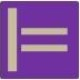## Reflections on Abstractions: Correctness and Completeness

Abstraction Awareness is about deeper understanding of abstraction, a concept so basic to human thinking. Subsequently, in strive for rigour, an earlier post on quality properties of models is compared to basic concepts of mathematical logic.

##### 1. Correct and Complete, simply putThe former post, gave a brief explanation what correct, complete, etc. means for models, as in the figure.  Where correct meant, no requirement in the model is wrong and thus every solution accepted by the customer (in C) is in accord with the model (in M) and complete is, no requirement in the model is missing and thus every solution according to the model (in M) is also accepted by the customer (in C).

##### 2. The concept of a Formal System

In mathematical logic a formal system (FS) is a triple of a language syntax, a set of axioms and a resolution mechanism, for example, the syntax of first order logic, the axioms of the theory of equivalence relations, and the |- resolution operator, such that theorems can be derived from the axioms.

A structure that obeys such a FS is said to be a model. For example, for my red and white socks, being of equal colour defines an equivalence-relation.

A FS has at least one model if, and only if, it is consistent, i.e. the axioms are not self-contradicting, and thus can be fulfilled.

So, consistency assumed, the axioms define a set of models A, as well as any theorem defines a set of models T, and thus proving a theorem (by the resolution operator) comes down to show that the models of the axioms A are a subset of the models of the theorem T.

##### 3. Correct and Complete, formally put

Now the above situation in a FS seems quite similar to that of a ‘customer system’: instead of written down axioms in some language, we have requirements, ‘hidden inside the head’ of the customer, that we have to explore by stating requirements (theorems). Instead of a resolution mechanism we have the customer itself, as a kind of oracle that answers our questions.

Thus, when a requirement is accepted by the customer it defines a superset of the accepted solutions (models), and hence is said to be correct. Correspondingly, if the requirements altogether define a subset of the accepted solutions (models), they are said to be complete. Thus, correct and complete, ensures that the stated and the accepted solutions are congruent (consistency assumed).

So long
|=

PS
Concerning ambiguity: if there is at most one model for the axioms in the FS, then together with consistency (at least one model), the model is said to be unique up to isomporphy (because maths is a structural science).